# Some thoughts (and simulation) on overfitting

## November 12, 2016

Reading time ~13 minutes

Overfitting is a common problem in data analysis. Some go as far as saying that “most of” published research is false (John Ionnadis); overfitting being one, maybe central, problem of it. In this post, we explore some aspects on the notion of overfitting.

Assume we have 10 metric variables v (personality/health/behavior/gene indicator variables), and, say, 10 variables for splitting up subgroups (aged vs. young, female vs. male, etc.), so 10 dichotomic variables. Further assume there are no association whatsoever between these variables. How likely is it we find something publishable? Apparently quite probably; but let’s give it a try.

Load some packages.

library(tidyverse)
library(corrr)
library(broom)
library(htmlTable)


# Simulated dataset 10x10

Make sup some uncorrelated data, 10x10 variables each $N \sim (0,1)$.

set.seed(123)
matrix(rnorm(100), 10, 10) -> v


Let’s visualize the correlation matrix.

v %>% correlate %>% rearrange %>% rplot(print_cor = TRUE)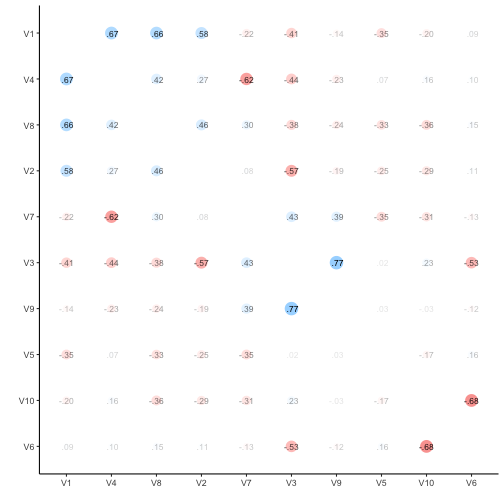v %>% correlate -> v_corr_matrix


So, quite some music in the bar. Let’s look at the distribution of the correlation coefficients.

v_corr_matrix %>%
select(-rowname) %>%
gather %>%
filter(complete.cases(.)) -> v_long

v_long %>%
mutate(r_binned = cut_width(value, 0.2)) %>%
ggplot +
aes(x = r_binned) +
geom_bar()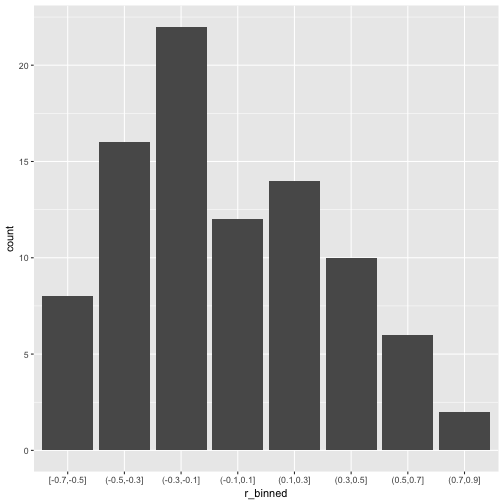What about common statistics?

library(psych)
describe(v_long$value)  ## vars n mean sd median trimmed mad min max range skew kurtosis ## X1 1 90 -0.03 0.36 -0.12 -0.05 0.35 -0.68 0.77 1.44 0.36 -0.68 ## se ## X1 0.04  Probably more stringent to just choose one triangle from the matrix, but I think it does not really matter for our purposes. Quite a bit of substantial correlation going on. Let’s look from a different angle. v_long %>% ggplot + aes(x = 1, y = value) + geom_violin(alpha = .3) + geom_jitter(aes(x = 1, y = value), width = .1) + xlab("") + geom_hline(yintercept = mean(v_long$value), linetype = "dashed", color = "#880011")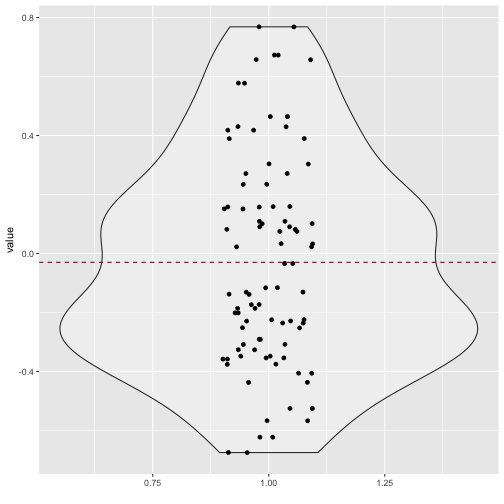## 100x100 dataset correlation

Now let’s scale up a bit and see whether the pattern stabilize.

set.seed(123)
matrix(rnorm(10^4), 100, 100) -> v2
v2 %>% correlate -> v2_corr_matrix

v2_corr_matrix %>%
select(-rowname) %>%
gather %>%
filter(complete.cases(.)) -> v2_long

v2_long %>%
mutate(r_binned = cut_width(value, 0.2)) %>%
ggplot +
aes(x = r_binned) +
geom_bar(aes(y = ..count../sum(..count..))) +
ylab("proportion")So, how many r’s are in the (0.1,0.3) bin?

library(broom)
v2_long %>%
mutate(r_binned = cut_width(value, 0.2)) %>%
group_by(r_binned) %>%
summarise(n_per_bin = n(),
prop_per_bin = round(n()/nrow(.), 2))

## # A tibble: 5 × 3
##      r_binned n_per_bin prop_per_bin
##        <fctr>     <int>        <dbl>
## 1 [-0.5,-0.3]        10         0.00
## 2 (-0.3,-0.1]      1594         0.16
## 3  (-0.1,0.1]      6776         0.68
## 4   (0.1,0.3]      1512         0.15
## 5   (0.3,0.5]         8         0.00


That’s quite a bit; 15% correlation in the bin (.1.3). Publication ahead!

# Subgroup analyses

Now, let’s look at our subgroup. For that purposes, we need actual data, not only the correlation data. Let’s come up with 1000 individuals, where he have 100 measurement (metric; N(0,1)), and 100 subgroup variables (dichotomic).

set.seed(123)
matrix(rnorm(1000*100), nrow = 1000, ncol = 100) -> v3
dim(v3)

##  1000  100

matrix(sample(x = c("A", "B"), size = 1000*100, replace = TRUE), ncol = 100, nrow = 1000) -> v4
dim(v4)

##  1000  100

v5 <- cbind(v3, v4)
dim(v5)

##  1000  200


Now, t-test battle. For each variable, compute a t-test; for each subgroup, compute a t-test. Do the whole shabeng (100*100 tests).

But, as a first step, let’s look at the first subgroup variable only.

v3 %>%
data.frame %>%
map(~t.test(.x ~ v4[, 1])) %>%
map(broom::tidy) %>%
map("p.value") %>%
unlist %>%
tibble -> v3_pvalues

v3_pvalues %>%
qplot(x = .) +
xlab("p value") +
geom_vline(xintercept = .05, color = "#880011", linetype = "dashed")

## Don't know how to automatically pick scale for object of type tbl_df/tbl/data.frame. Defaulting to continuous.

## stat_bin() using bins = 30. Pick better value with binwidth.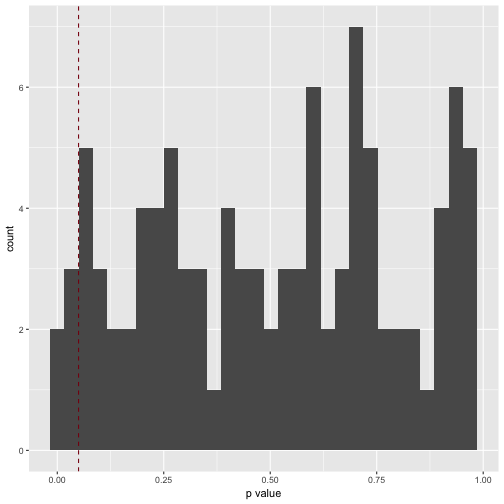How many significant p values resulted (should be 5%, ie 5 of 100)?

v3_pvalues %>%
filter(. < .05)

## # A tibble: 5 × 1
##            .
##        <dbl>
## 1 0.01350926
## 2 0.04115726
## 3 0.01064116
## 4 0.04251559
## 5 0.02505199


Which is exactly what we found.

p_list <- vector(length = 100, mode = "list")
i <- 1

for (i in 1:100){

v3 %>%
data.frame %>%
map(~t.test(.x ~ v4[, i])) %>%
map(broom::tidy) %>%
map("p.value") %>%
unlist %>%
tibble -> p_list[[i]]

}

# str(p_list)  #tl;dr

p_df <- as.data.frame(p_list)
names(p_df) <- paste("V", formatC(1:100, width = 3, flag = "0"), sep = "")


Let’s try a giant plot with 100 small multiples.

p_df %>%
gather %>%
ggplot(aes(x = value)) +
geom_histogram() +
facet_wrap(~key, ncol = 10)

## stat_bin() using bins = 30. Pick better value with binwidth.Well impressive, but that histogram matrix does not serve much. What about a correlation plot as above, only giantesque…

p_df %>% correlate %>% rplot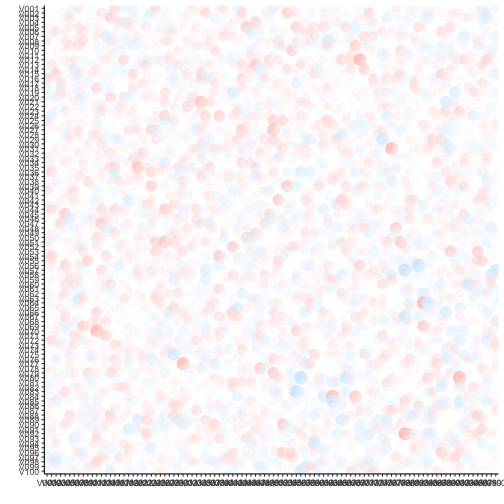Hm, of artis values (in 10000 years). Maybe better plot a histogram of the number of signifinat p-values ( <. 05).

p_df %>%
gather %>%
dplyr::filter(value < .05) %>%
qplot(x = value, data = .) +
xlab("p value")

## stat_bin() using bins = 30. Pick better value with binwidth.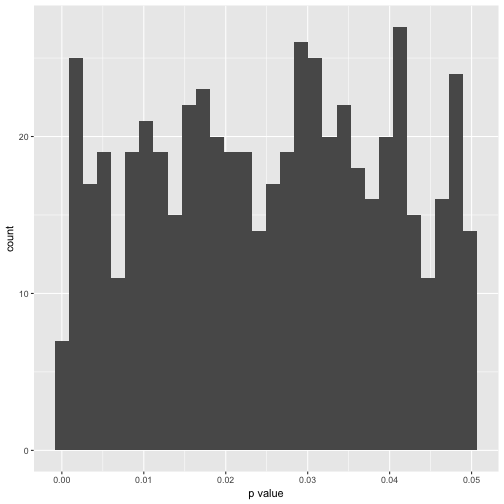In exact numbers, what’s the percentage of significant p-values per variable?

p_df %>%
gather %>%
group_by(key) %>%
filter(value < .05) %>%
summarise(n = n()) -> p_significant

htmlTable(p_significant)

key n
1 V001 5
2 V002 4
3 V003 8
4 V004 7
5 V005 4
6 V006 4
7 V007 4
8 V008 5
9 V009 3
10 V010 5
11 V011 6
12 V012 8
13 V013 7
14 V014 4
15 V015 13
16 V016 7
17 V017 8
18 V018 5
19 V019 7
20 V020 4
21 V021 10
22 V022 3
23 V023 5
24 V024 4
25 V025 7
26 V026 9
27 V027 4
28 V028 7
29 V029 5
30 V030 3
31 V031 4
32 V032 9
33 V033 8
34 V034 8
35 V035 4
36 V036 2
37 V037 11
38 V038 8
39 V039 2
40 V040 10
41 V041 6
42 V042 3
43 V043 5
44 V044 2
45 V045 8
46 V046 2
47 V047 8
48 V048 7
49 V049 2
50 V050 5
51 V051 4
52 V052 10
53 V053 5
54 V054 3
55 V055 3
56 V056 5
57 V057 3
58 V058 2
59 V059 1
60 V060 8
61 V062 6
62 V063 5
63 V064 3
64 V065 12
65 V066 4
66 V067 6
67 V068 2
68 V069 9
69 V070 6
70 V071 7
71 V072 8
72 V073 8
73 V074 8
74 V075 5
75 V076 4
76 V077 5
77 V078 4
78 V079 3
79 V080 4
80 V081 6
81 V082 9
82 V083 12
83 V084 5
84 V085 3
85 V086 6
86 V087 7
87 V088 4
88 V089 6
89 V090 6
90 V091 6
91 V092 7
92 V093 4
93 V094 5
94 V095 2
95 V096 10
96 V097 7
97 V098 6
98 V099 5
99 V100 2

Distribution of significant p-values.

p_significant %>%
qplot(x = n, data = .)

## stat_bin() using bins = 30. Pick better value with binwidth.Summary stats of significant p-values.

p_df %>%
gather %>%
group_by(key) %>%
filter(value < .05) %>%
summarise(n_p = n(),
mean_p = mean(value),
median_p = median(value),
sd_p = sd(value, na.rm = TRUE),
IQP_p = IQR(value),
min_p = min(value),
max_p = max(value)) %>%
map(mean) %>% as.data.frame -> p_summary_tab

## Warning in mean.default(.x[[i]], ...): argument is not numeric or logical:
## returning NA


Here comes the table of the mean over all 100 variables of the typical statistics.

p_summary_tab %>%
select_if(is.numeric) %>%
round %>%
htmlTable

key n_p mean_p median_p sd_p IQP_p min_p max_p
1 6 0 0 0 0 0

In sum, overfitting - mistakenly taking noise as signal - happened often. Actually, as often as is expected by theory. But, not knowing or not minding theory, one can easily be surprised by the plethora of “interesting findings” in the data. In John Tukey’s words (more or less): “Torture the data for a long enough time and it will tell you anything”.

### New bar stacking with ggplot 2.2.0

Recently, ggplot2 2.2.0 was released. Among other news, stacking bar plot was improved. Here is a short demonstration.Load libraries`...… Continue reading

#### Crashkurs zur Erstellung von Barplots für Umfrage-Daten

Published on November 13, 2016

#### Preparing and plotting survey results

Published on November 10, 2016